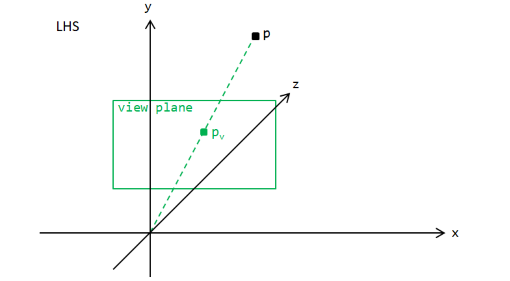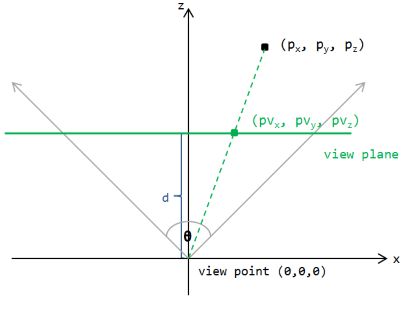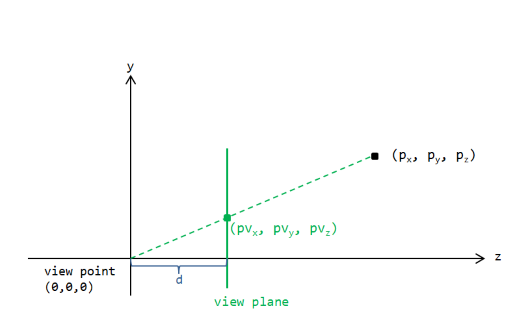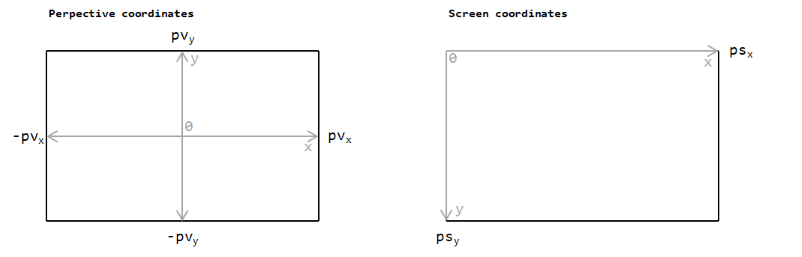Simplistic fixed camera to perspective screen transformation

# 1. Introduction

Here you are reading my personal notes I made for myself while trying to understand the so-called camera-to-perspective and subsequent perspective-to-screen transformation.
So what's this all about? This is one of the fundamentals of every 3D engine. Assume you have a point in the three-dimensional space, defined by its coordinates x, y and z, and you want to project it onto your screen, depending on your current location and direction of view. How do you do this? If you have asked yourself this question, then you are at the right location!

I always like to start new topics in a very primitive manner, to really get a basic understanding (or feeling) for this topic. Therefore, following definitions and simplifications are used in the following explanations:

• A LHS (left handed coordinate system) used, so the x-axis goes to right, y-axis to top and z-axis 'into the screen'.
• The camera, also called view point, is located at the origin of the coordinate system. It is looking straight down the z-axis.
• Rotation, viewing frustum and clipping planes are not considered - only the fundamental process to calculate the perspective and screen coordinates is examined.Above diagram shows the used left handed coordinate system.
The camera is located at the origin (0, 0, 0) and has the direction ("looks at") straight down the positive z-axis.
The view plane is an imaginery plane which is parallel to the x-axis and y-axis and perpendicular to the z-axis. A rectangluar portion of this plane with a defined width and height forms the screen. The view plane has distance d from the origin.

The goal is to project point p from three dimensional space onto the view plane and calculate the final two dimensional point on the screen.
The whole process is split into two steps:

1. Perspective transformation: This projects the point (based on camera coordinates) onto the view plane. Note that the perspective coordinate is not equal to the coordinate on our screen.
The camera point p is transformed to perspective coordinate pv.
2. Screen transformation: Transforms the perspective coordinate to the actual screen coordinate.
The perspective coordinate pv is transformed to screen coordinate ps.

# 2. Perspective coordinates

The easiest approach to retrieve the formulas for perspective projection is to analyze the geometry for the 2D case, once for the x-z plane and once for the y-z plane and finally combine the retrieved information to get the overall projection formulas.

## 2.1 Projection in X-Z plane and Y-Z planeOn the left side, the x-z plane of the coordinate system is shown. This can be imagined as we look at it from the top, right along from the positive y-axis to the origin.
The location of point pv can be calculated easily with the basic geometry, using the proportions of similar triangles:
pvx / px = pvz / pz
Rearraning the formula to separate pvx and knowing that pvz = d results in:
pvx = d * px / pz

On the right side, the y-z plane is shown. Similar to the case above, the projected point onto the view plane is calculated using the proportions of similar triangles:
pvy / py = pvz / pz
Rearraning the formula to separate pvy and knowing that pvz = d results in:
pvy = d * py / pz

In a nutshell, our camera-to-perspective transformation is:
pvx = d * px / pz
pvy = d * py / pz
where p is the 3D point in camera coordinates, pv the projected point p onto the view plane and d is the distance from the origin to the view plane.

Well, unfortunately it is not that easy, as the distance d and the aspect ratio ar. So let's see ...

## 2.2 View plane distance d

The question arises how to choose the distance d to the view plane? The answer is actually quite simple: You can choose it whatever you want. Of course, it must somehow match with the logical unit used with for your camera coordinates.
In practice, one of the following two methods are the simplested to use:

• Set d = 1. The perspective coordinates are then called normalized perspective coordinates.
• Calculate d from the field of view to match the screen size. I call then the perspective coordinates as view plane perspective coordinates. This is my preferred option.

Before examining both perspective projection types, at first the field of view (FOV) needs to be shortly discussed:The field of view (FOV) is the extent of the observable game world, normally measured as an angle. In the diagram above, the FOV Θ is 90 degrees (90°). Obviously, there is the following geometric relation between FOV Θ, distance d of the view plane and width w of the view plane:
tan(Θ/2) = (w/2)/d.

Note that for a FOV of 90°, tan(Θ/2) is 1. This implies that w/2 = d.

## 2.3 Normalized perspectice coordinates (d = 1)

An easy way is to strictly define d = 1. On the one hand, this simplifies the perspective transformation formulas to pvx = px / pz and pvy = py / pz.
On the other hand, when assuming the common field of view of 90°, both sides of the triangle have equal length, thus d = w/2.

Note: For further discussion of normalized perspectice coordinates, only a FOV of 90° is used. Of course it is possible to use another FOV, but then the range of the calculated perspectice coordinates will change which needs to be considered by an additional factor in the screen transformation. For simplicity, this is not considered here.

Using a FOV of 90°, the perspective coordinates fall into the range [-1, 1]. This is apparant when considering the px and pz coordinates:
If |px| = pz, then the point lies directly on the field of view lines (grey lines in diagram above), so the point is mappted to pvx = -1 if px = -1 respectively to pvx = 1 if px = 1.
If |px| > pz, then the point lies outside the field of view and is not projected onto the view plane.
If |px| < pz, then the point is inside the field of view cone so |px| / pz < |-1|.

The same applies for py, however only when using square projection view with equal width and height. In general, screens have a non-square area where normally the width is larger than the height. Here comes the aspect ratio (ar) into play which is the ratio between screen width and screen height and is defined as ar = width / height.
For example, a screen with size 1920 x 1080 has an aspect ratio of ar = 1920 / 1080 = 1,77 (or 16:9).
So the y-coordinates are not mapped into range [-1, 1] but to [-1/ar, -1/ar]. This can be achieved by multiplying pvy with ar during the perspective transformation.
So considering the aspect ratio, the perspective transformation formulas are:
pvx = px / pz and
pvy = py * ar / pz.

Aspect ratio:
The aspect ratio can be tricky. It is important to consider it at any step when using normalized coordinates. I think it's easier to handle it as factor in the perspective transformation than in a later step.

## 2.4 View plane perspective coordinates

Another way is to calculate the distance d so that the view plane size matches with the target screen size. So distance d is calculated from the screen width and the FOV Θ.

Above, the formula tan(Θ/2) = (w / 2) / d was derived. Solving for d results in:
d = 0.5 * w / tan(Θ/2).
The remaining perspective transformation is the same as already derived above:
pvx = px * d / pz
pvy = py * d / pz.

Aspect ratio:
Interestingly, the aspect ratio needs not to be considered here when using view plane perspective coordinates. I am not absolutely sure why it is like that, but I assume considering the screen size for calculating the distance and no multiplication is required in the screen transformation, you get the aspect ratio handling for free :-)

# 3. Screen coordinates

After having calculated the perspective coordinates from the camera coordinates, the final step is to retrieve the actual screen coordinates. Following two diagrams show the needed transformation:There are two points that needs to be considered:
Point 1: The y-axis is inverted. It goes from top to bottom on the screen instead of from bottom to top as in the perspective coordinate system.
Point 2: The center (0,0) is the middle of the view plane in the perspective coordinate system while it is in the top left corner in the screen coordinate system.

The exact transformation depends again on the fact if normalized perspective coordinates (d = 1) or view plane perspective coordinates (arbitrary d to map view plane) are used. Note also that the screen width ranges from [0, ScreenWidth - 1] and screen height from [0, ScreenHeight -1 ].

## 3.1 Screen coordinates from normalized perspective coordinates

The perspective coordinates ranges needs to be mapped to screen coordinates ranges:
X: [-1, 1]           -----> [0, ScreenWidth - 1]
Y: [-1 * ar, 1 * ar] -----> [0, ScreenHeight - 1]

In x-direction, at first one is added to x to change the range from [-1, 1] to [0, 2]. Then x is multiplied by half of the screen width including rounding, resulting in:
x = (x + 1) * ((ScreenWidth - 1) / 2)
= (x + 1) * (0.5*ScreenWidth - 0.5).

In y-direction, it is not necessary to handle the aspect ratio range value explicitely - it is only important to consider the aspect ratio once in the process, and this has been already done in the perspective transformation. The y coordinate has to be inverted, this can be done by y = (ScreenHeight - 1) - y. Then, like in the x-direction case, one is added to shift the range starting at zero and the coordinate need to be scaled by half of the screen height considering rounding. This results in:
y = (ScreenHeight - 1) - (y + 1) * ((ScreenHeight - 1) / 2)
= (ScreenHeight - 1) - (y * ((ScreenHeight - 1) / 2) + ((ScreenHeight - 1) / 2))
= (ScreenHeight - 1) - (y * (0.5*ScreenHeight - 0.5) + (0.5*ScreenHeight - 0.5)
= ScreenHeight - 1 - y * (0.5*ScreenHeight - 0.5) - 0.5*ScreenHeight + 0.5
= - y * (0.5*ScreenHeight - 0.5) + (0.5*ScreenHeight + 0.5).

## 3.2 Screen coordinates from view plane perspective coordinates

The perspective coordinates ranges needs to be mapped to screen coordinates ranges:
X: [-(ScreenWidth - 1)/2, (ScreenWidth - 1)/2]   -----> [0, ScreenWidth - 1]
Y: [-(ScreenHeight - 1)/2, (ScreenHeight - 1)/2] -----> [0, ScreenHeight - 1]

In x-direction, this is a simple translation by half of the screen width, thus:
x = x + ((ScreenWidth - 1) / 2)
= x + (0.5*ScreenWidth - 0.5)

In y-direction, the translation is by half of the screen height, so y = y + ((ScreenHeight - 1) / 2). Further, the y coordinate has to be inverted, this can be done by y = (ScreenHeight - 1) - y. Put everthing together, this results in:
y = (ScreenHeight - 1) - (y + ((ScreenHeight - 1) / 2))
= ScreenHeight - 1 - y - ((ScreenHeight - 1) / 2)
= ScreenHeight - 1 - y - 0.5*ScreenHeight + 0.5
= 0.5*ScreenHeight - 0.5 - y   = -y + ((ScreenHeight - 1) / 2).

# 4. Summary

Summarized, the camera - perspective - screen transformation is as follows, depending on the used projection coordinate mode.

Normalized perspective coordinates:
• Camera-to-perspective transformation (p -> pv):
pvx = px / pz
pvy = py * ar / pz

• Perspective-to-screen transformation (pv -> ps):
psx = (pvx + 1) * (0.5*ScreenWidth - 0.5)
psy = -py * (0.5*ScreenHeight - 0.5) + (0.5*ScreenHeight + 0.5)

View plane perspective coordinates:

• Camera-to-perspective transformation (p -> pv):
pvx = px * d / pz
pvy = py * d / pz

• Perspective-to-screen transformation (pv -> ps):
psx = pvx + (0.5*ScreenWidth - 0.5)
psy = -pvy + (0.5*ScreenHeight + 0.5)

That's it! Hope it was interesting for you and have fun and learned something! Keep coding!

Sunshine, October 2020

#### History

• 2020/10/01: Initial version.